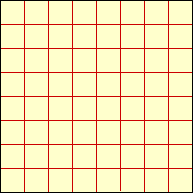Quandaries and Queries I have a question for you. I have never, ever heard of furlongs, and my question is "1 mile=8 furlongs, how many cubic furlongs in a cubic mile?" And can you please explain to me how you got it. Thank you, Hi, The only place where I hear the term furlong used is in horse racing. One of the standard lengths for a race is one-eighth of a mile, which is a furlong. Suppose that you have a square that is one mile by one mile and you want to divide it into squares that are one furlong by one furlong.Since each mile gets divided into 8 furlongs the square mile gets divided into 64 square furlongs. What happens if you start with a cubic mile? Penny Go to Math Central